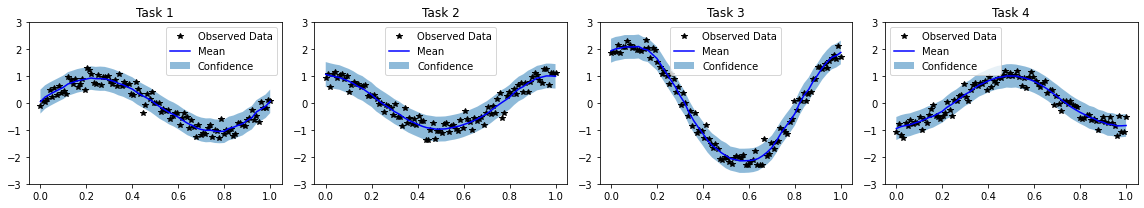# Deep GPs and DSPPs w/ Multiple Outputs¶

## Introduction¶

In this example, we will demonstrate how to construct deep GPs that can model vector-valued functions (e.g. multitask/multi-output GPs).

This tutorial can also be used to construct multitask deep sigma point processes by replacing DeepGPLayer/DeepGP/DeepApproximateMLL with DSPPLayer/DSPP/DeepPredictiveLogLikelihood.

:

import os
import torch
import tqdm
import math
import gpytorch
from torch.nn import Linear
from gpytorch.means import ConstantMean, LinearMean
from gpytorch.kernels import MaternKernel, ScaleKernel
from gpytorch.variational import VariationalStrategy, CholeskyVariationalDistribution, \
LMCVariationalStrategy
from gpytorch.distributions import MultivariateNormal
from gpytorch.models.deep_gps import DeepGPLayer, DeepGP
from gpytorch.mlls import DeepApproximateMLL, VariationalELBO
from matplotlib import pyplot as plt

smoke_test = ('CI' in os.environ)
%matplotlib inline


### Set up training data¶

In the next cell, we set up the training data for this example. We’ll be using 100 regularly spaced points on [0,1] which we evaluate the function on and add Gaussian noise to get the training labels.

We’ll have four functions - all of which are some sort of sinusoid. Our train_targets will actually have two dimensions: with the second dimension corresponding to the different tasks.

:

train_x = torch.linspace(0, 1, 100)

train_y = torch.stack([
torch.sin(train_x * (2 * math.pi)) + torch.randn(train_x.size()) * 0.2,
torch.cos(train_x * (2 * math.pi)) + torch.randn(train_x.size()) * 0.2,
torch.sin(train_x * (2 * math.pi)) + 2 * torch.cos(train_x * (2 * math.pi)) + torch.randn(train_x.size()) * 0.2,
-torch.cos(train_x * (2 * math.pi)) + torch.randn(train_x.size()) * 0.2,
], -1)

train_x = train_x.unsqueeze(-1)


## Structure of a multitask deep GP¶

The layers of a multitask deep GP will look identical to the layers of a single-output deep GP.

:

# Here's a simple standard layer

class DGPHiddenLayer(DeepGPLayer):
def __init__(self, input_dims, output_dims, num_inducing=128, linear_mean=True):
inducing_points = torch.randn(output_dims, num_inducing, input_dims)
batch_shape = torch.Size([output_dims])

variational_distribution = CholeskyVariationalDistribution(
num_inducing_points=num_inducing,
batch_shape=batch_shape
)
variational_strategy = VariationalStrategy(
self,
inducing_points,
variational_distribution,
learn_inducing_locations=True
)

super().__init__(variational_strategy, input_dims, output_dims)
self.mean_module = ConstantMean() if linear_mean else LinearMean(input_dims)
self.covar_module = ScaleKernel(
MaternKernel(nu=2.5, batch_shape=batch_shape, ard_num_dims=input_dims),
batch_shape=batch_shape, ard_num_dims=None
)

def forward(self, x):
mean_x = self.mean_module(x)
covar_x = self.covar_module(x)
return MultivariateNormal(mean_x, covar_x)


The main body of the deep GP will look very similar to the single-output deep GP, with a few changes.

Most importantly - the last layer will have output_dims=num_tasks, rather than output_dims=None. As a result, the output of the model will be a MultitaskMultivariateNormal rather than a standard MultivariateNormal distribution.

There are two other small changes, which are noted in the comments.

:

num_tasks = train_y.size(-1)
num_hidden_dgp_dims = 3

def __init__(self, train_x_shape):
hidden_layer = DGPHiddenLayer(
input_dims=train_x_shape[-1],
output_dims=num_hidden_dgp_dims,
linear_mean=True
)
last_layer = DGPHiddenLayer(
input_dims=hidden_layer.output_dims,
linear_mean=False
)

super().__init__()

self.hidden_layer = hidden_layer
self.last_layer = last_layer

# We're going to use a ultitask likelihood instead of the standard GaussianLikelihood

def forward(self, inputs):
hidden_rep1 = self.hidden_layer(inputs)
output = self.last_layer(hidden_rep1)
return output

def predict(self, test_x):

# The output of the model is a multitask MVN, where both the data points
# and the tasks are jointly distributed
# To compute the marginal predictive NLL of each data point,
# we will call to_data_independent_dist,
# which removes the data cross-covariance terms from the distribution.
preds = model.likelihood(model(test_x)).to_data_independent_dist()

return preds.mean.mean(0), preds.variance.mean(0)



### Training and making predictions¶

This code should look similar to the DGP training code.

:

model.train()
mll = DeepApproximateMLL(VariationalELBO(model.likelihood, model, num_data=train_y.size(0)))

num_epochs = 1 if smoke_test else 200
epochs_iter = tqdm.notebook.tqdm(range(num_epochs), desc="Epoch")
for i in epochs_iter:
output = model(train_x)
loss = -mll(output, train_y)
epochs_iter.set_postfix(loss=loss.item())
loss.backward()
optimizer.step()



:


# Make predictions
model.eval()
test_x = torch.linspace(0, 1, 51).unsqueeze(-1)
mean, var = model.predict(test_x)
lower = mean - 2 * var.sqrt()
upper = mean + 2 * var.sqrt()

# Plot results[ ]: